# Practice calculations for a relativistic speed object

• Lamdbaenergy
In summary, at 99.99999999999999 percent of the speed of light, an object weighing 150,000 kilograms gets a relativistic mass increase of 183,648,173,214,437,391 kilograms, therefore getting a relativistic energy of 16,505,474,674,203,172,344,215,046,082,011,484 joules (3,944,903,124,809,553,619 megatons.) This energy is far larger than the necessary energy needed to completely destroy a planet like the Earth, which is about over 53 quadrillion megatons worth of

#### Lamdbaenergy

Effects of a 150,000 kilogram object launched at Earth, just below the speed of light: 99.99999999999999 percent 'c' speed.

The object weighing 150,000 kilograms gets a relativistic mass increase of 183,648,173,214,437,391 kilograms, therefore getting a relativistic energy of 16,505,474,674,203,172,344,215,046,082,011,484 joules (3,944,903,124,809,553,619 megatons.) This energy is far larger than the necessary energy needed to completely destroy a planet like the Earth, which is about over 53 quadrillion megatons worth of TNT (mean-estimate.) At nearly 4 quintillion megatons, this object has enough energy to create a literal planet-killing explosion upon impact, doing a lot more than just sterilizing the surface of the Earth of all life.
Kinetic energy in this object increases in a relativistic way to 16,505,474,674,189,691,016,533,993,817,411,484 joules (3,944,903,124,806,331,505 megatons.) And that means the relative kinetic energy is pretty much equal to the overall relative energy inside the object now.

The material slams into the Earth's surface in an inconceivably short amount of time and, since it's moving so fast, the particles in the atmosphere are practically frozen in time. All of the particles nitrogen, oxygen, water vapor, carbon dioxide, and whatever else in the air is hit with so much force in such a short time scale, that nuclear fusion occurs. The explosive energy release from the object is about 4 quintillion megatons, which is about 7,400 - 7,500 percent the power needed to cause total planetary devastation. Whatever continent the object strikes at its velocity is immediately annihilated as an explosion the likes of which the world has never seen erupts and completely destroys the Earth. Whether or not the Earth would become a new asteroid belt orbiting around the Sun, I do not yet know.

Were the calculations accurate? Please correct me if I'm wrong on some parts! Thanks!

I am reminded of the story Mark Twain told about the dowager on a sea voyage who spotted an iceberg in the distance and asked the captain what would happen if the ship and the iceberg collided. The captain, looking very somber, said gravely "The iceberg, madam, would continue onward as though nothing had happened".

It just doesn't seem to me that the Earth is strong enough to slow down something traveling that fast. I could well be wrong, but I think it would just punch a hole, with the circumference of the hole having fusion effects and that plus the resulting shock-wave shattering the Earth, as the object continued onward as though nothing had happened.

I realize that my thinking might be entirely off because I'm thinking in classical physics terms, like a bullet going though paper, instead of quantum terms, like a proton hitting lead and spewing particles.

Vsauce3's video talked about Superman punching you in the face at the speed of light. The explosion would be equivalent to 45 megatons. Your face wouldn't block Superman's punch and neither would the Earth, you're saying? Or maybe Superman would punch through you and keep moving as the explosion happened? Confusing but thanks for alerting me to that!

Of course the Earth is not strong enough to stop this object, as it has two orders of magnitude more energy than the gravitational binding energy of the Earth (assuming you did your calculations right, I did not redo them myself). This means, even if this object ejected every part of the Earth to infinity, it still wouldn't slow down significantly. What will likely happen is it will collide with the Earth and form a jet of material moving at very high speeds in the direction this object was originally moving. I'm guessing the Earth will not be completely destroyed (although the surface probably will be) because it's hard to distribute all that energy over the entire Earth.

But we'd still prefer Superman throw a light speed punch at 99 percent rather than this object at 99.99999999999999 percent! I don't think any alien civilization will ever be able to create some relativistic death weapon that throws objects at planets at those velocities. Would they? And thanks for alerting me to how the Earth wouldn't stop the material at the surface! I can't believe I didn't think about that!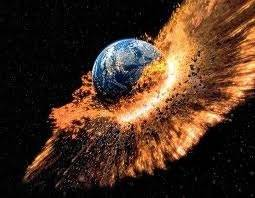Lamdbaenergy said:
16,505,474,674,189,691,016,533,993,817,411,484 joules

Were the calculations accurate?

Accurate? They certainly are precise.

Let me start out with a wiki reference for a concept I'm going to use extensively in my answer, rapidity:
http://en.wikipedia.org/w/index.php?title=Rapidity&oldid=638135825

If the gamma-factor that multiples energy is about 10^12 as stated , since gamma = cosh(rapidity), the rapidity should be about 28.3, using a moderately sane level of precision.

However, since rapidity = tanh(v/c) = tanh(beta) , and we can approximate tanh(x) for large x as ##1 - e^{-2x}##, I get that for such a large gamma, the velocity should be different from c by less than 1 part in 10^24. And it looks like the original expression for v is different from c by about 1 part in 10^16. So there's apparently probably a problem here in the original calculation, reverse-calculating from the specified gamma doesn't give the specified velocity.

It would make the most sense to me to specify the gamma factor and not the velocity, since that's more of what is of interest, but I don't know what the OP's intent was. Probably just to check their calculations. If that's the case, I'm not getting the same results (While I wouldn't rule out that I made an error, I don't appear to be getting the same answers).

However, I'm not too sure where to proceed from there in an attempt to answer the rest of the question. If we take for example the case where the rapidity was 28.3, we can imagine a frame where the Earth and the impactor are both moving with a rapidity of 14.15, since rapidities add. This corresponds to a gamma factor of about 700,000, a bit different than my intuitive guess of the square root , but not by so much to make me suspect an error.

For comparison, proton-proton collisions at Cern have a gamma factor of about 4,000 or so, for protons - less than that I think for lead ions (which are also planned to be collided in Cern, and would probably better match the original problem). So we're out of the ballpark of Cern-style collision energies in this hypothetical scenario.

I was hoping that there be a way to use the CERN cross-sections to figure out a bit about what happens in the center-of-rapidity frame, at least how many collisions occured. Critically, though, I'm not sure how to define a "collision" for this cacluation, except that I'm looking for a collision to involve a significant conversion of the kinetic energy into some explosion and/or jet of particles, rather than say a "glancing blow" that releases only some minuscule fraction of the available energy.

I'd also have to say that I think "fusion" as per the original post would be unlikely, using the CERN analogy I'd expect a quark-gluon plasma like they expect to study in the lead ion collisions. Rather than fusing things together, I imagine you'd be breaking them into pieces so small that even protons wouldn't survive intact. But I'm beginning to step out of the area I know here.

pervect said:
It would make the most sense to me to specify the gamma factor and not the velocity, since that's more of what is of interest, but I don't know what the OP's intent was. Probably just to check their calculations. If that's the case, I'm not getting the same results (While I wouldn't rule out that I made an error, I don't appear to be getting the same answers).
I wouldn't trust the original calculation unless it was done by someone with some awareness of the pitfalls of high-precision computer arithmetic - there are going to be a lot of bits in the significands of some of the intermediate results and subtracting values very near to 1.0 from 1.0 under those circumstances is asking for trouble.

Equations I used:

Relative kinetic energy = (mc^2/(sqrt(1 - v2/c2))) - mc^2

Relative energy = (mc^2/(sqrt(1 - v2/c2)))

Relative mass = (m/(sqrt(1 - v2/c2)))

Is this where I might be wrong? I just knew the equations and plugged the numbers in and got the results in my paragraph. Could the equations be more complicated than I thought?

Lamdbaenergy said:
Effects of a 150,000 kilogram object launched at Earth, just below the speed of light: 99.99999999999999 percent 'c' speed.

Equations I used:

Relative kinetic energy = (mc^2/(sqrt(1 - v2/c2))) - mc^2

Relative energy = (mc^2/(sqrt(1 - v2/c2)))

Relative mass = (m/(sqrt(1 - v2/c2)))

Is this where I might be wrong? I just knew the equations and plugged the numbers in and got the results in my paragraph. Could the equations be more complicated than I thought?

Given ##v/c = \beta = 1 - \epsilon## , counting the digits I get ##\epsilon = 10^{-16}## using scientific notation, (see http://en.wikipedia.org/w/index.php?title=Scientific_notation&oldid=638667511 if the term is unfamiliar, though I hope it is familiar) which I'll use throughout.

Lets re-write the equation for ##\gamma = 1 / \sqrt{1 - \beta^2}## in terms of ##\epsilon##, where we define ##\beta = 1 - \epsilon## as above. Then

##\gamma = 1 / \sqrt{1 - \left( 1 - \epsilon \right) ^2} = 1 / \sqrt{1 - 1 + 2 \epsilon - \epsilon^2} \approx 1 / \sqrt{2 \epsilon}##

Because ##2 \epsilon >> \epsilon^2## we can neglect the later term, justifying the approximation in the last step above. Plugging into the equations I get:

##\gamma \approx 7.1*10^8## and "relativistic mass" = ##\gamma m## = ##1.5*10^5## times ##7.1*10^8 \approx1.1*10^{13}## = 11,000,000,000,000, which is considerably different from your answer, though the equations do look correct.

This corresponds to a rapidity of 21.1 if you want to go that route.

Here is what I did for mass; I used the equation below.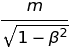I'm just going to have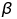represent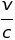Plugging in the numbers, I just ended up with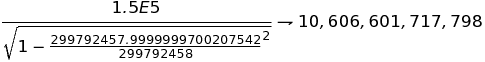So I realize where I messed up. I originally made the velocity precisely 299792457.9999999999999999 instead of 299792457.9999999700207542! That's where the confusion came from all along. Now I can redo the calculations...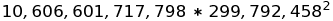That should give you about 953 octillion joules, rather than over 16 decillion.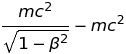This should give me a kinetic energy that it pretty close to the relativistic energy. So the object would actually be carrying an energy of barely 230 trillion megatons rather than 4 quintillion. Luckily, this energy is still too far below the required amount needed to destroy the Earth, and it would very likely just end up penetrating right through the crust and mantle, creating a shockwave that would horribly disfigure a good portion of the planet's surface, but not destroy it all.